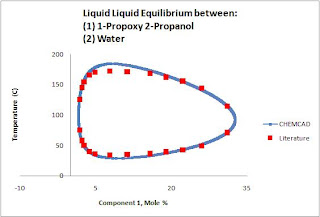## Tuesday, October 13, 2009

### The Challenge of Unusual LLE

When friends and colleagues ask me what the CHEMCAD technical support group does, the best answer I have is: "We help chemical engineers address their challenges." This runs the gamut from simple to hard problems, and might include pushing for changes in CHEMCAD itself. One challenge we resolved was how to model some very non-ideal LLE systems in CHEMCAD.

The binary system of 1-propoxy 2-propanol and water has unusual behavior. There is both an upper and a lower critical solution temperature to the liquid-liquid region. Modeling this type of system in CHEMCAD proved to be quite a challenge.

What do we mean by upper and lower critical solution temperatures? As you increase temperature, you can form a second liquid phase. Keep increasing temperature, and the two liquid phases will coalesce back into a single liquid phase. A phase diagram for this behavior shows a closed ovoid.

If you wanted to model this, you would start by asking the following questions:
- Can CHEMCAD model liquid-liquid separation?
- Can CHEMCAD model this unusual liquid-liquid behavior?
- How do we (as users and engineers) inspect and adjust the simulator's model results for this problem?

You can use CHEMCAD to model a heterogeneous azeotrope—that is, a system where two liquid phases separate from each other. You can plot binary LLE diagrams, or the ternary 'binodal plot' that many of us remember from college.

For this simulation, we chose the NRTL K-value model. The NRTL model enables you to calculate γi from binary interaction parameters (BIPs). You can use γi to solve yi P = γi xi pisat. Normally this is used to calculate vapor-liquid equilibrium (Ki=yi/xi), but an optional modification to NRTL enables it to model liquid-liquid equilibrium. It's important to note that using NRTL with LLE will predict a second liquid phase only if the BIPs (from the CHEMCAD database or your work) will result in LLE.

It’s always wise to check what a K-value model is calculating, because sometimes you might need to adjust the settings for the model you’re using. One set of BIPs doesn't necessarily cover all conditions; the mathematical models aren't powerful enough to handle "all phenomena" with a single set of parameters.

In this case, using the Plot > Binary LLE and Plot > TPXY commands in CHEMCAD showed that the NRTL model did not match experimental data for the liquid-liquid separation.

To address this, we tried to quickly regress new BIPs from the literature data (Thermophysical > Regress BIPs). Regressing the LLE data did give us marginally better results, but we could not match the 'closed circle' seen in the experimental data for this unusual system.

Next, we decided to calculate the activity coefficient of each data point, and then regress a multi-parameter BIP from the activity coefficients. The results are shown below:CHEMCAD now fits the experimental data quite well! We are now able to show the effects of having both an upper and a lower critical solution temperature. Using a sensitivity study and a flash unit makes it possible to move into (and back out of) the LLE region on a flowsheet.